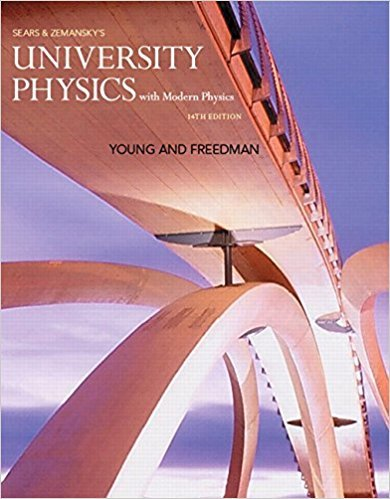×
×

# A 0.800-kg ornament is hanging by a 1.50-m wire when theISBN: 9780321973610 228

## Solution for problem 8.45 Chapter 8

University Physics with Modern Physics (1) | 14th Edition

• Textbook Solutions
• 2901 Step-by-step solutions solved by professors and subject experts
• Get 24/7 help from StudySoup virtual teaching assistantsUniversity Physics with Modern Physics (1) | 14th Edition

4 5 0 412 Reviews
15
2
Problem 8.45

A 0.800-kg ornament is hanging by a 1.50-m wire when the ornament is suddenly hit by a 0.200-kg missile traveling horizontally at 12.0 m>s. The missile embeds itself in the ornament during the collision. What is the tension in the wire immediately after the collision?

Step-by-Step Solution:
Step 1 of 3

Physics Week 8 Chapter 26: Direct Current Circuits Kirchhoff’s Law 1 (Voltage Law) In a closed conducting path, the sum of potential differences is zero ΣV = 0 Ɛ = V 1 V + 2 3 - Ɛ + V 1 V + 2 = 0 3 Kirchhoff’s Law 2 (Current Law) Sum of currents entering is equal to the sum of currents leaving in a circuit junction ΣI = 0 Resistors in Series R eqR...

Step 2 of 3

Step 3 of 3

##### ISBN: 9780321973610

Unlock Textbook Solution